×#### Thank you for registering.

One of our academic counsellors will contact you within 1 working day.

Click to Chat

1800-1023-196

+91-120-4616500

CART 0

• 0

MY CART (5)

Use Coupon: CART20 and get 20% off on all online Study Material

ITEM
DETAILS
MRP
DISCOUNT
FINAL PRICE
Total Price: Rs.

There are no items in this cart.
Continue Shopping• Complete JEE Main/Advanced Course and Test Series
• OFFERED PRICE: Rs. 15,900
• View Details

```Chapter 4: Cubes and Cube Roots Exercise – 4.2

Question: 1

Find the cubes of:

(i) – 11    (ii) – 12     (iii) – 21

Solution:

(i) Cube of – 11 is given as: (-11)3 = – 11 x – 11 x – 11 = – 1331

Thus, the cube of 11 is (-1331).

(ii) Cube of – 12 is given as: (-12)3 = – 12 x – 12 x – 12 = – 1728

Thus, the cube of – 12 is (- 1728).

(iii) Cube of – 21 is given as:

(- 21)3 = – 21 x – 21 x – 21 = – 9261

Thus the cube of – 21 is (- 9261).

Question: 2

Which of the following numbers are cubes of negative integers?

(i) – 64

(ii) – 1056

(iii) – 2197

(iv) – 2744

(v) – 42875

Solution:

In order to check if a negative number is a perfect cube, first check if the corresponding positive integer is a perfect cube. Also, for any positive integer m, – m3 is the cube of – m.

(i)  On factorizing 64 into prime factors, we get:

64 = 2 x 2 x 2 x 2 x 2 x 2

On grouping the factors in triples of equal factors, we get:

64 = {2 x 2 x 2} x {2 x 2 x 2}

It is evident that the prime factors of 64 can be grouped into triples of equal factors and no factor is left over. Therefore, 64 is a perfect cube. This implies that – 64 is also a perfect cube.

Now, collect one factor from each triplet and multiply, we get: 2 x 2= 4

This implies that 64 is a cube of 4. Thus, – 64 is the cube of -4.

(ii) On factorising 1056 into prime factors, we get:

1056 = 2 x 2 x 2 x 2 x 2 x 3 x 11

On grouping the factors in triples of equal factors, we get:

1056 = {2 x 2 x 2}x 2 x 2 x 3 x 11

It is evident that the prime factors of 1056 cannot be grouped into triples of equal factors such that no factor is left over.

Therefore, 1056 is not a perfect cube. This implies that – 1056 is not a perfect cube as well.

(iii) On factorising 2197 into prime factors, we get:

2197 =13 x 13 x 13

On grouping the factors in triples of equal factors, we get:

2197 = {13 x 13 x13}

It is evident that the prime factors of 2197 can be grouped into triples of equal factors and no factor is left over. Therefore, 2197 is a perfect cube. This implies that – 2197 is also a perfect cube.

Now, collect one factor from each triplet and multiply, we get 13. This implies that 2197 is a cube of 13. Thus, -2197 is the cube of – 13.

(iv) On factorizing 2744 into prime factors, we get:

2744 = 2 x 2 x 2 x 7 x 7 x 7

On grouping the factors in triples of equal factors, we get: 2744 = {2 x 2 x 2} x {7 x 7 x 7}

It is evident that the prime factors of 2744 can be grouped into triples of equal factors and no factor is left over. Therefore, 2744 is a perfect cube. This implies that – 2744 is also a perfect cube.

Now, collect one factor from each triplet and multiply, we get:

2 x 7 = 14

This implies that 2744 is a cube of 14.

Thus, – 2744 is the cube of – 14.

(v) On factorizing 42875 into prime factors, we get:

42875 = 5 x 5 x 5 x 7 x 7 x 7

On grouping the factors in triples of equal factors, we get:

42875 = {5 x 5 x 5} x {7 x 7 x 7}

It is evident that the prime factors of 42875 can be grouped into triples of equal factors and no factor is left over. Therefore, 42875 is a perfect cube.

This implies that – 42875 is also a perfect cube.

Now, collect one factor from each triplet and multiply, we get: 5 x 7 = 35

This implies that 42875 is a cube of 35. Thus, – 42875 is the cube of – 35.

Question: 3

Show that the following integers are cubes of negative integers. Also find the integer whose cube is the given integer.

(i) – 5832   (ii) – 2744000

Solution:

In order to check if a negative number is a perfect cube, first check if the corresponding positive integer is a perfect cube. Also, for any positive integer in, – m3 is the cube of – m.

(i) On factorising 5832 into prime factors, we get:

5832 = 2 x 2 x 2 x 3 x 3 x 3 x 3 x 3 x 3

On grouping the factors in triples of equal factors, we get:

5832 = 2 x 2 x 2 x 3 x 3 x 3 x 3 x 3 x 3

It is evident that the prime factors of 5832 can be grouped into triples of equal factors and no factor is left over. Therefore, 5832 is a perfect cube.

This implies that -5832 is also a perfect cube.

Now, collect one factor from each triplet and multiply, we get:

2 x 3 x 3 = 18

This implies that 5832 is a cube of 18.

Thus, – 5832 is the cube of – 18.

(ii) On factorising 2744000 into prime factors, we get:

2744000 = 2 x 2 x 2 x 2 x 2 x 2 x 5 x 5 x 5 x 7 x 7 x 7

On grouping the factors in triples of equal factors, we get:

2744000 = {2 x 2 x 2} x {2 x 2 x 2} x {5 x 5 x 5} x {7 x 7 x 7}

It is evident that the prime factors of 2744000 can be grouped into triples of equal factors and no factor is left over. Therefore, 2744000 is a perfect cube. This implies that – 2744000 is also a perfect cube.

Now, collect one factor from each triplet and multiply, we get: 2 x 2 x 5 x 7 = 140

This implies that 2744000 is a cube of 140. Thus, – 2744000 is the cube of – 140.

Question: 4

Find the cube of:

(i) 7/9

(ii) – (8/11)

(iii) 12/7

(iv) –(13/8)

(v) 2(2/5)

(vi) 3(1/4)

(vii) 0.3

(viii) 1.5

(ix) 0.08

(x) 2.1

Solution: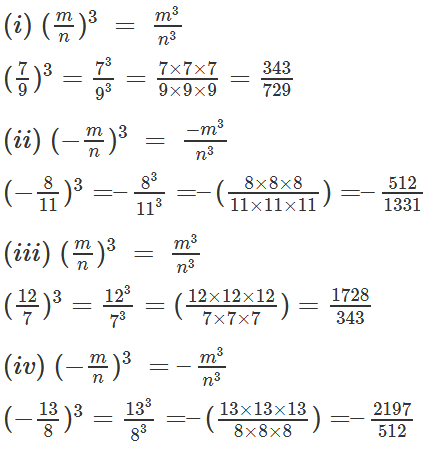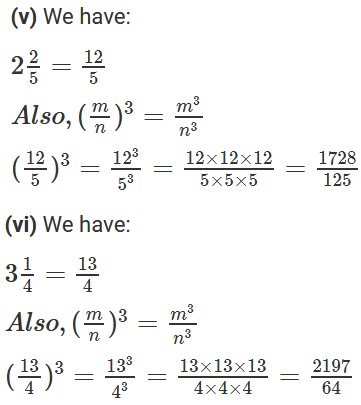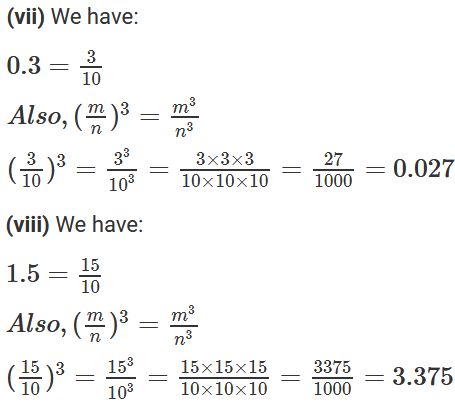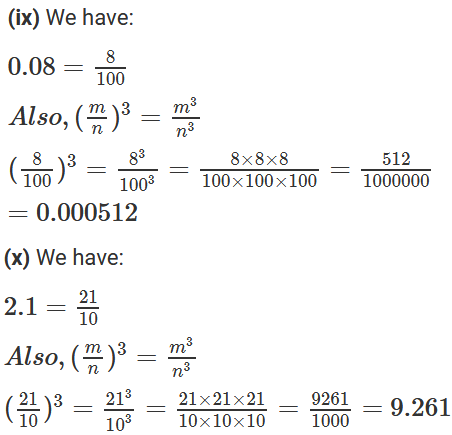Question: 5

Find which of the following numbers are cubes of rational numbers?

(i) 27/64

(ii) 125/128

(iii) 0.001331

(iv) 0.04

Solution: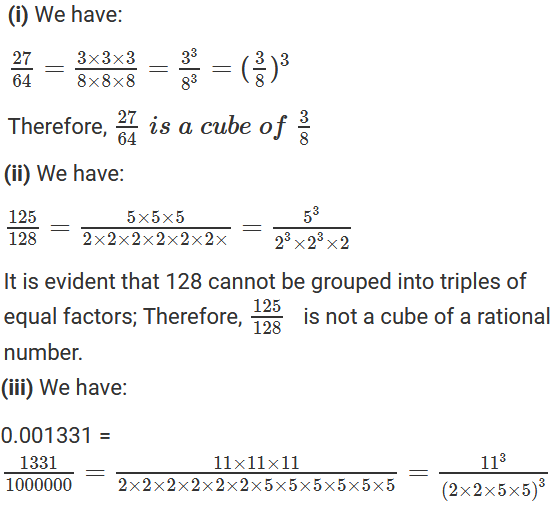```### Course Features

• 728 Video Lectures
• Revision Notes
• Previous Year Papers
• Mind Map
• Study Planner
• NCERT Solutions
• Discussion Forum
• Test paper with Video Solution